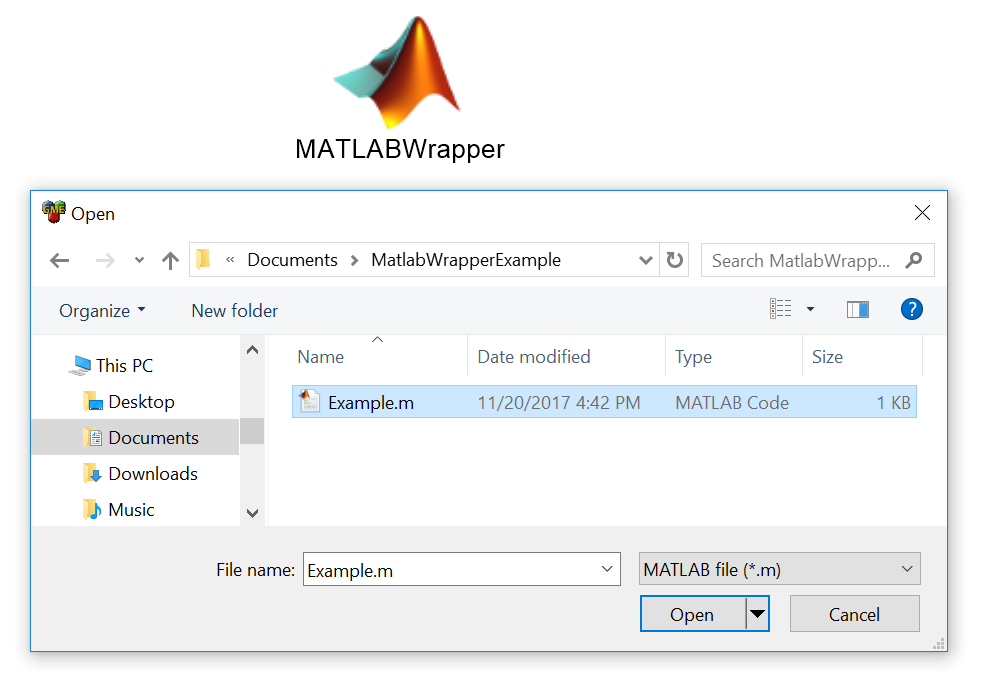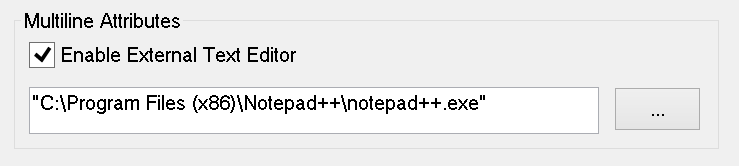# MATLAB Wrappers¶

MATLAB Wrappers allow the user to execute custom MATLAB scripts and functions in the context of a PET. There are two types of MATLAB scripts that can be integrated: Function Files and Bare Files. The MATLAB Wrapper determines the type by examining the script file.

Currently, only MATLAB version 2015a and later are supported.

## Function Files¶

In a Function File you define a function with the same name as the script filename and this becomes the entry point for the script. There are a few restrictions in the current implementation:

• Only scalar (double) type values are allowed as inputs and outputs.
• There can be more than one function declared in the script, but the wrapper will only use as an entry point the function with the same name as the script filename. This name is case-sensitive and must match exactly.

Below is a Function File example of a MATLAB Wrapper script:

Example.m
```function [sum, product] = Example(x, y, z)
sum = x + y + z
product = x * y * z
end
```

## Bare Files¶

In a Bare File you define the inputs and outputs of the script by a set of specially-formatted comments at the beginning of the file. These comments allow you to define the data type of all the inputs and outputs.

Below is a Bare File example of a script that doubles a number of different types of inputs:

Double.m
```% variable: output1 double output
% variable: output2 double[] output
% variable: output3 string output
% variable: output4 string[] output
% variable: input1 double input
% variable: input2 double[] input
% variable: input3 string input
% variable: input4 string[] input

output1 = input1 * 2
output2 = input2 * 2
output3 = strcat(input3, input3)
output4 = [input4, input4]
```

You cannot define functions in a Bare File style MATLAB integration file; however, you can call other function files that you have defined.

### MATLAB Data Type Conversion¶

OpenMETA uses the Python OpenMDAO framework to execute PETs. Since the data passed between analysis blocks is managed by Python, the table below describes the conversions that occur when data is passed into or out of a MATLAB Wrapper block.

Python to MATLAB to Python
Boolean Logical Boolean
Int N/A 
List of Ints N/A 
Numpy Int Array Double Array Numpy Float Array 
Float Double Float 
List of Floats N/A 
Numpy Float Array Double Array Numpy Float Array
String N/A 
List of Strings Cell Array of Strings List of Strings
Numpy Array of Strings N/A 
Unicode Char Unicode
List of Unicodes Cell Array of Unicodes List of Unicodes
Dictionary Struct Dictionary 
  (1, 2, 3, 4, 5) These types are not allowed to be passed into MATLAB Wrapper analysis blocks.
  Integers in an array will be converted to floats upon passing through a MATLAB Wrapper analysis block.
  All doubles in MATLAB are essentially a one-by-one array (1x1), so the framework automatically unwraps all one-by-one arrays to a single float value as they are passed to the next analysis block. E.g. A 1x1 Numpy Array will become a double in MATLAB and will result in a double in OpenMDAO when it is passed to the next analysis block.
  Structs in MATLAB can only accept fieldnames that meet the following three criteria: Start with a letter Contain only letters, numbers, and/or the underscore character Must be no longer than `namelengthmax` (currently 63) characters Although Python can handle arbitrary strings as the keys in dictionaries, you must meet these criteria if you are going to pass the dictionaries to a MATLAB Wrapper block.

For examples of the conversion see the “MatlabConversions” PET in the Analysis Blocks project in the Openmeta Examples And Templates repository.

## Configuring MATLAB Wrappers¶

To add a Matlab Wrapper to a PET, simply drag the Matlab Wrapper icon from the Part Browser and onto the PET canvas.To configure a MATLAB Wrapper, double-click on the MATLAB Wrapper and use the Open dialogue to select the MATLAB script to be added to the PET.A MATLAB Wrapper loaded with a MATLAB script

If you subsequently want to make changes to the script, you can do so directly from GME by using the Edit () button. Alternatively, if you make edits to your script outside of GME, you can reload the script using the Reload () button.

Tip

You can change the default editor in GME by opening Tools ‣ Options and checking Enable External Text Editor.Configuring Notepad++ as the External Editor